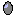#Reproduction

The reproduction symbol is used to specify the rate of creation of new instances of a population submodel by each existing instance. It thus differs from the migration symbol, which specifies the total rate of creation of new instances. It belongs to the group of symbols known as channels. The symbol's icon represents an egg.

## How to add a Reproduction symbol

The reproduction symbol only has meaning in the context of a population submodel. Therefore, it makes sense to construct a population submodel first, then add the reproduction symbol to it. This is not strictly necessary: you can add the reproduction symbol first, then construct a submodel around it, then make the submodel into a population submodel, but it is better practice to construct the population submodel first.

## Rules

• The reproduction symbol can receive influence arrows from anywhere in the model, including from within the same submodel. This information can then be used to calculate the current value for the rate of creation of new instances of the population. As with all other relationships within a population submodel (except for the migration symbol), influences coming from within the same submodel relate to the properties of the same instance. So, for example, an influence from a variable "bodyweight" to the reproduction symbol indicates that it is each individual's body weight that influences its own rate of reproduction.
• You can include as many reproduction symbols as you like. Biologically it is unusual to have more than one method for reproduction (though some organisms reproduce asexually and sexually at different stages of their life cycle), but it is perfectly legal as far as Simile is concerned.
• It is possible to draw an influence arrow from the reproduction symbol to another element. The usual use of this is with the channel_is( ) function. The value of the reproduction symbol is the value of its equation.

## Interpretation

The reproduction symbol captures the concept that, in many biological situations, the production of new individuals by those already in the population - reproduction - is an important mechanism for increasing population size. Moreover, the ability of an individual to reproduce will depend on its own characteristics: its age or weight, for example.

As with the migration symbol, Simile needs to resolve the fact that the value for reproduction is a floating point number, while new individuals can only be created one-by-one. The method it uses to do this is similar to that used for migration, and essentially involves the use of the reproduction term to contribute fractions of an individual to an accumulator: when the accumulator exceeds a whole number, then that number of new instances for the submodel are created, and the accumulator is reduced by the number of instances created.

There is, however, an important issue that the designers of Simile had to address. Should there be one accumulator for the whole population, or one for each of the current set of instances? In the former case, if you had five instances, each with a reproduction value of 0.1, then one new individual would be created every 2 time units. In the latter case, for the same settings, you would get no new instances for ten time units, then five would be created at the same time. The first approach seems more attractive, but suffers from a fatal flaw: it assumes that the parentage of newly-created individuals is irrelevant. This would severely restrict the modelling you could do: in particular, it would rule out modelling evolution, since that requires some concept of (biological) inheritance, which in turn means that each individual needs to know who its parent(s) are. Also, the second approach gives the same behaviour as the first if the value for reproduction is treated stochastically (e.g. as a probability), rather than as a precise deterministic contribution until you have enough credit to make one individual. Therefore, in Simile, each individual accumulates its own credit, starting with a random fraction, until it has sufficient to make one new individual.

Like migration channels, reproduction channels can be discrete event valued. If the event that triggers a reproduction event is outside the population submodel, then when it occurs, all individuals in the population will reproduce, subject in each case to the equation of the reproduction channel having a positive value. Alternatively the triggering event can be inside the population submodel, in which case only the individuals in which the event occurs will reproduce.

As in the case of the migration symbols, the accumulators are initialized to random fractions between 0 and 1. This ensures that if the reproduction rates are the same in a lot of individuals, the new ones do not all appear in the same time step but in a random pattern with no pre-determined peaks or troughs. If this non-deterministic behaviour is not required, the technique described for deterministic migration can be used to eliminate it.